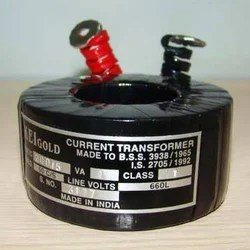# What is CT & PT

## Current Transformers (CT)

Current transformers are generally used to measure currents of high magnitude. These transformers step down the current to be measured, so that it can be measured with a normal range ammeter. A Current transformer has only one or very few number of primary turns. The primary winding may be just a conductor or a bus bar placed in a hollow core (as shown in the figure). The secondary winding has large number turns accurately wound for a specific turns ratio. Thus the current transformer steps up (increases) the voltage while stepping down (lowering) the current.
Now, the secondary current is measured with the help of an AC ammeter. The turns ratio of a transformer is NP / NS = IS / IPOne of the common application of a current transformer is in a ‘Digital Clamp Meter’.
Generally, current transformers are expressed in their primary to secondary current ratio. A 100:5 CT would mean the secondary current of 5 amperes when primary current is 100 amperes. The secondary current rating is generally 5 amperes or 1 ampere, which is compatible with standard measuring instruments.

### Potential Transformer (PT)

Potential transformers are also known as voltage transformers and they are basically step down transformers with extremely accurate turns ratio. Potential transformers step down the voltage of high magnitude to a lower voltage which can be measured with standard measuring instrument. These transformers have large number of primary turns and smaller number of secondary turns.
A potential transformer is typically expressed in primary to secondary voltage ratio. For example, a 600:120 PT would mean the voltage across secondary is 120 volts when primary voltage is 600 volts.